# IB DP Chemistry: SL复习笔记11.1.1 Index of Hydrogen Deficiency

### Index of Hydrogen Deficiency

• The degree of unsaturation or index of hydrogen deficiency provides information about the number of double or triple bonds in a molecule
• The IHD is the number of hydrogen molecules, H2, needed to convert the molecule to the corresponding saturated, non-cyclic molecule
• There are two ways to solve IHD problems. One way is to draw the structure and identify rings and double and triple bonds, counting each one as an IHD value of 1.
• The second way is to use a formula,
• For a compound containing CxHyIHD= (2x+2-y)/2
• This is a little complicated, since for the formula to work you need to:
• ignore O and S
• count halogens as hydrogen
• add one C and one H for every nitrogen in the formula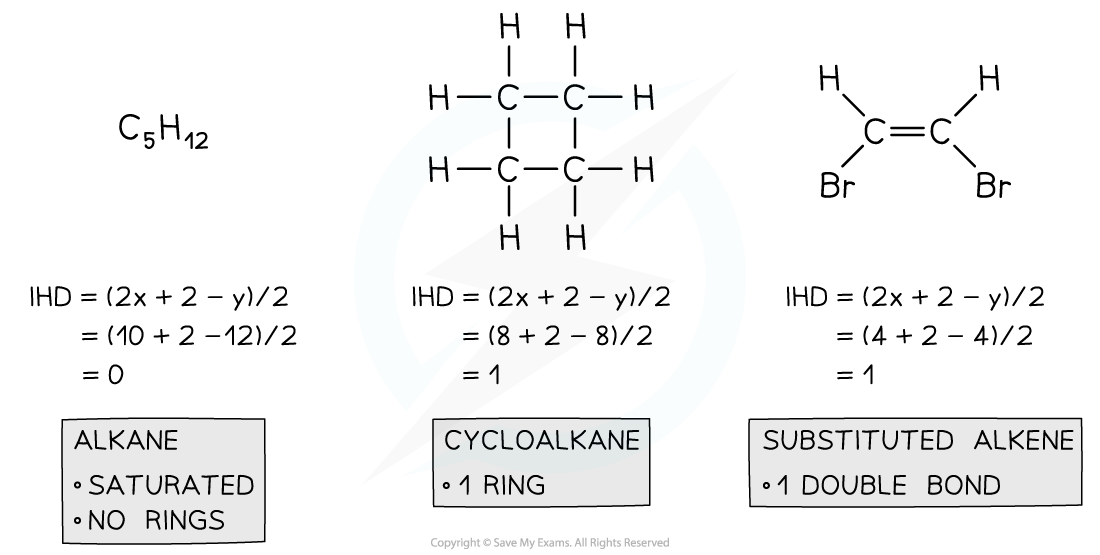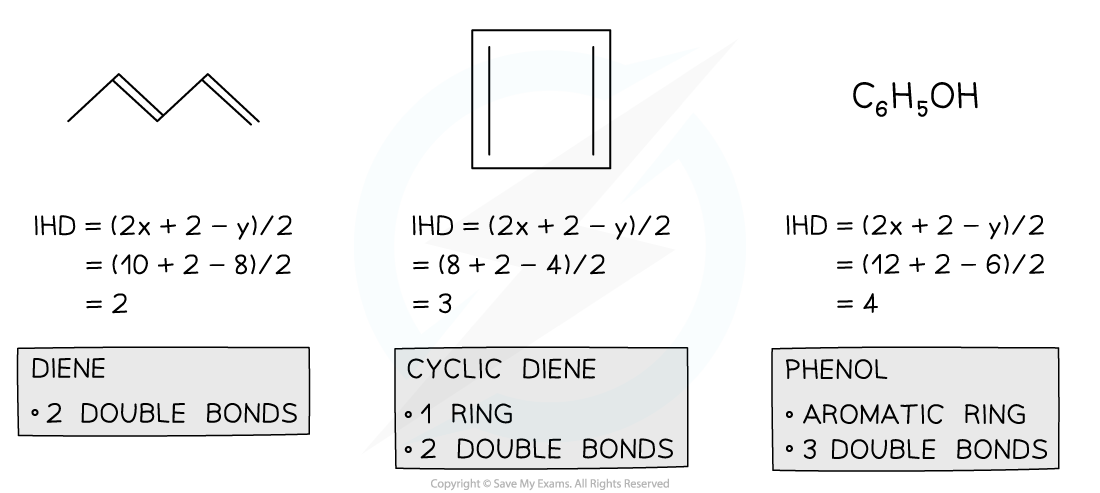Index of Hydrogen Deficiency Examples

#### Worked Example

Deduce the Index of Hydrogen Deficiency in ethyne, C2H2, methyl benzene, C6H5CH3, and propanone, CH3COCH3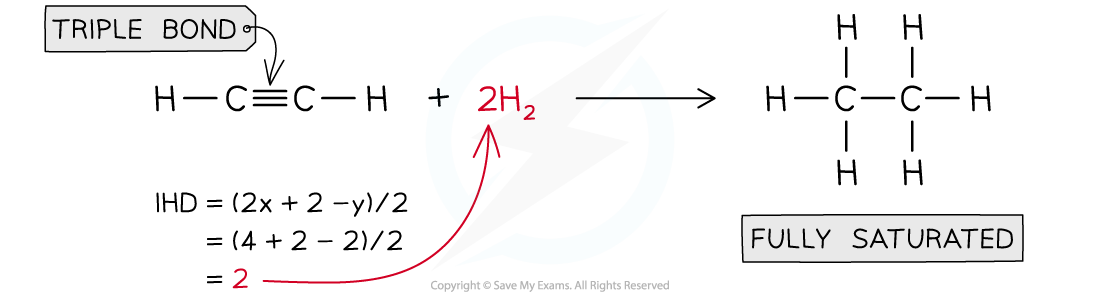Answer 2: Methylbenzene, C6H5CH3, IHD= 4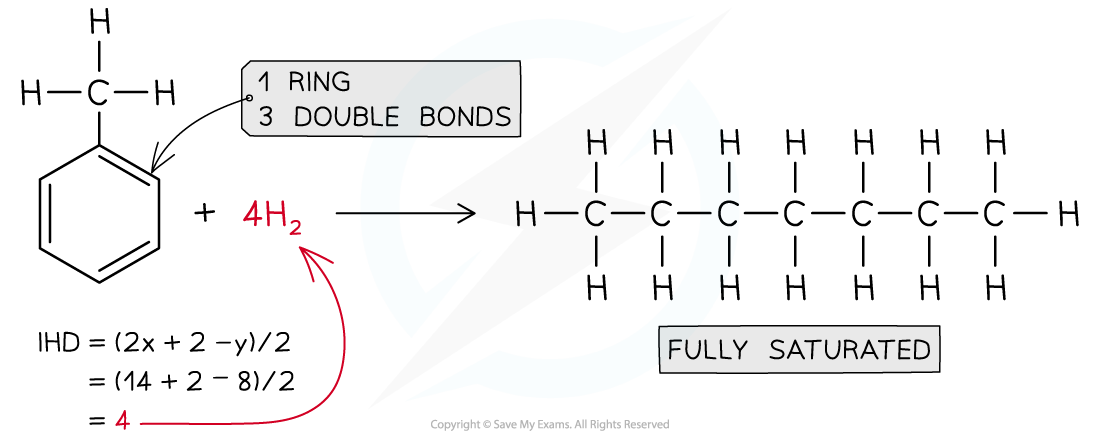Answer 3: Propanone,CH3COCH3  IHD = 1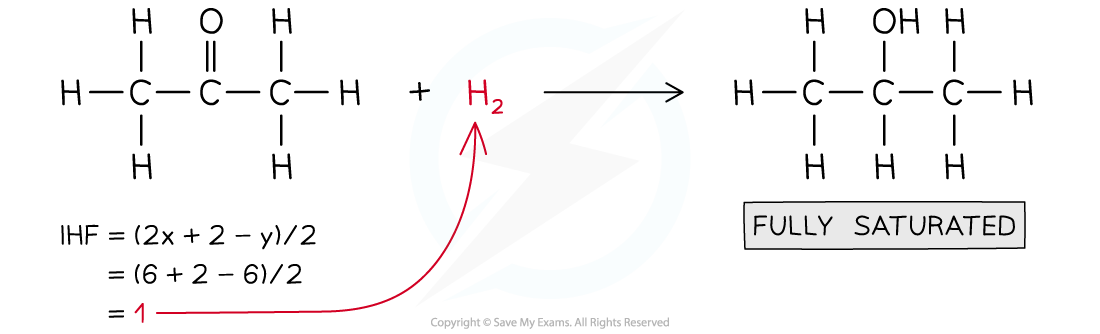#### Exam Tip

Drawing out the structure is a much faster way to solve IHD problems, but using the formula is helpful when you are struggling to draw the structure